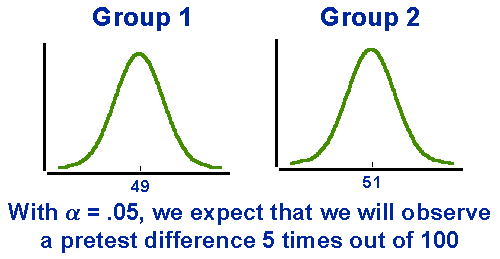Probabilistic Equivalence

# What is Probabilistic Equivalence?

What do I mean by the term probabilistic equivalence? Well, to begin with, I certainly don’t mean that two groups are equal to each other. When we deal with human beings it is impossible to ever say that any two individuals or groups are equal or equivalent. Clearly the important term in the phrase is “probabilistic”. This means that the type of equivalence we have is based on the notion of probabilities. In more concrete terms, probabilistic equivalence means that we know perfectly the odds that we will find a difference between two groups. Notice, it doesn’t mean that the means of the two groups will be equal. It just means that we know the odds that they won’t be equal. The figure shows two groups, one having a mean of 49 and the other with a mean of 51. Could these two groups be probabilistically equivalent? Certainly!We achieve probabilistic equivalence through the mechanism of random assignment to groups. When we randomly assign to groups, we can calculate the chance that the two groups will differ just because of the random assignment (i.e., by chance alone). Let’s say we are assigning a group of first grade students to two groups. Further, let’s assume that the average test scores for these children for a standardized test with a population mean of 50 were 49 and 51 respectively. We might conduct a t-test to see if the means of our two randomly assigned groups are statistically different. We know – through random assignment and the law of large numbers – that the chance that they will be different is `5 out of 100` when we set our significance level to `.05` (i.e., alpha = `.05`). In other words, 5 times out of every 100, when we randomly assign two groups, we can expect to get a significant difference at the `.05` level of significance.

When we assign randomly, the only reason the groups can differ is because of chance assignment because their assignment is entirely based on the randomness of assignment. If, by chance, the groups differ on one variable, we have no reason to believe that they will automatically be different on any other. Even if we find that the groups differ on a pretest, we have no reason to suspect that they will differ on a posttest. Why? Because their pretest difference had to be a chance one. So, when we randomly assign, we are able to assume that the groups do have a form of equivalence. We don’t expect them to be equal. But we do expect that they are “probabilistically” equal.